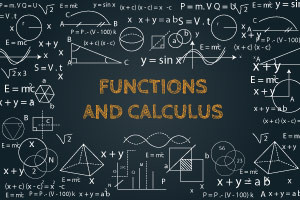Strand 5 Leaving Certificate Higher Level Functions and Calculus - Revised

Gain a better understanding of the concepts of function, differentiation calculus, Trapezoidal rule, and more.

Calculus
Free Course
A function is defined as the relation from a set of inputs to a set of possible outputs where each input is related to exactly one output. It will teach you about quadratic graphs, cubic graphs, and exponential graphs. You will learn how to plot graphs, sketch parabola, and translate functions. You will also look into differentiation calculus and integral calculus. This course is the final strand in Phase 3 of the new Project Maths Course.

3-4 Hours

Yes

Yes

Yes
• PublisherDescription

This course begins by teaching you how to plot quadratic graphs, reciprocal graphs, and exponential graphs from a table of values. You will learn how to match equations and sketches and look into the sketching of a parabola using completion of the square. Completing the square is the process where you change the form of the equation so that the left side is a perfect square trinomial. You will study the full method of completing the square, as well as learn about matching quadratic and exponential functions and graphs.

You will then look into the translation of functions using F(X-A). This course will teach you how to sketch functions in the X direction and in the Y-direction. You will study the discriminant, which is the part of the quadratic formula underneath the square root symbol b²-4ac and states whether there are two solutions, one solution, or no solutions to an equation. You will also cover inverse and bijective functions as well as differential calculus and integration calculus.

Functions and differentiation are used in real life to help us understand rates of increase and decrease. Upon the completion of this course, you will have a clearer understanding of the rules of differentiation, the basic integration of algebraic, trigonometrical, and exponential functions, the use of integration to find an area, and the Trapezoidal Rule. So, check out the course and easily learn the applications of calculus in the real world, today.

Start Course Now

Learning Outcomes

Having completed this course students will be able to:

• Identify how to plot Quadratic, cubic, reciprocal and exponential graphs from a table of values
• Identify how to translate or stretch a function along either the x or y axis
• Identify an inverse function and a bijective function
• Recognise how to calculate the gradient of a curve
• Calculate the differentiation of several different types of formulas and equations
• Identify the chain rule, the product rule and the quotient rule
• Calculate the turning points of a curve
• Use differentiation to calculate the displacement, velocity and acceleration, of an object
• Discuss the relationships between different equations/functions and their differentials
• Discuss what basic integration is and calculate it
• Define and calculate definite integration
• Calculate the area under a curve with integration

Certification

All Alison courses are free to enrol, study and complete. To successfully complete this Certificate course and become an Alison Graduate, you need to achieve 80% or higher in each course assessment. Once you have completed this Certificate course, you have the option to acquire official Certification, which is a great way to share your achievement with the world. Your Alison Certification is:

Ideal for sharing with potential employers - include it in your CV, professional social media profiles and job applications
An indication of your commitment to continuously learn, upskill and achieve high results
An incentive for you to continue empowering yourself through lifelong learning

Alison offers 3 types of Certification for completed Certificate courses:

Digital Certificate - a downloadable Certificate in PDF format, immediately available to you when you complete your purchase
Certificate - a physical version of your officially branded and security-marked Certificate, posted to you with FREE shipping
Framed Certificate - a physical version of your officially branded and security-marked Certificate in a stylish frame, posted to you with FREE shipping